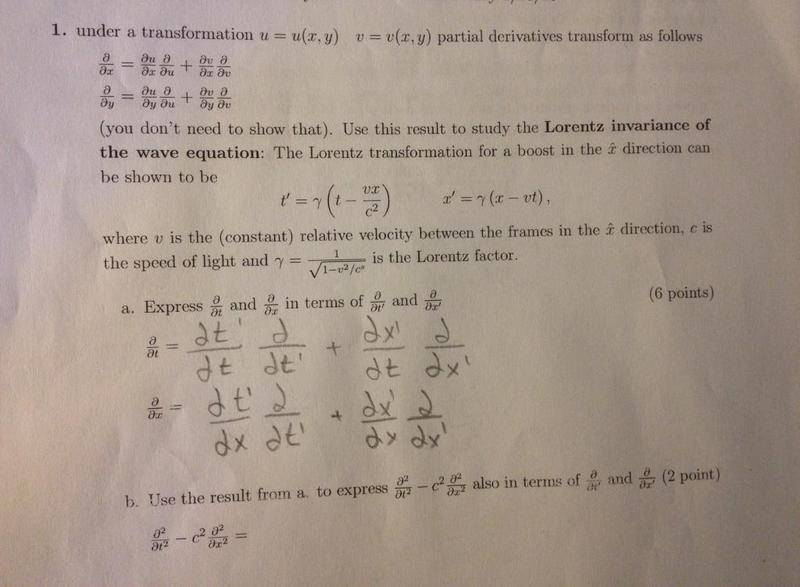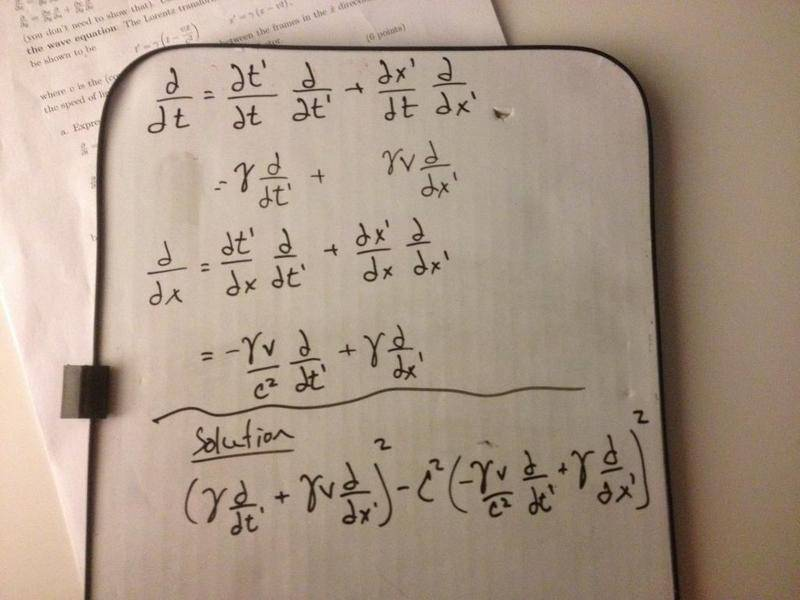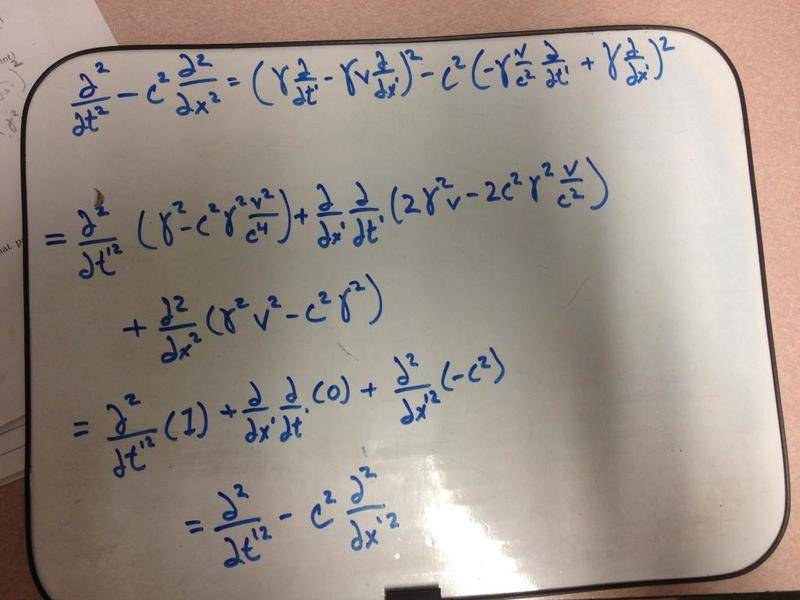# Partial derivatives transformation

• nmsurobert

## Homework Statement## Homework Equations

included in the first picture

## The Attempt at a Solutioni feel confident in my answer to part "a". i pretty much just did what the u and v example at the top of the page did. but for part "b" i tried to distribute and collect like terms and what not and i ended up with a huge mess. so i can anyone tell me if I am on the right track. and that the end product should like look a huge mess of terms.

thanks!

##\frac{\partial^2}{\partial t^2} \neq \left(\frac{\partial}{\partial t}\right)^2##
You have to apply the derivative twice.

so i figured out a solution and i thought i would add it here in case someone googling for information stumbles upon this thread.i get from the second step to the third step (where i am left only with derivatives and a c^2) using the definition of gamma given to me and some rigorous algebra.
took me a solid two days to figure this out.